Professor Murray

Sine and Cosine Values of Special Angles

Slide Duration:

Table of Contents

Section 1: Trigonometric Functions
Angles

39m 5s

Intro
0:00
Degrees
0:22
Circle is 360 Degrees
0:48
Splitting a Circle
1:13
Radians
2:08
Circle is 2 Pi Radians
2:31
One Radian
2:52
Half-Circle and Right Angle
4:00
Converting Between Degrees and Radians
6:24
Formulas for Degrees and Radians
6:52
Coterminal, Complementary, Supplementary Angles
7:23
Coterminal Angles
7:30
Complementary Angles
9:40
Supplementary Angles
10:08
Example 1: Dividing a Circle
10:38
Example 2: Converting Between Degrees and Radians
11:56
Example 3: Quadrants and Coterminal Angles
14:18
Extra Example 1: Common Angle Conversions
-1
Extra Example 2: Quadrants and Coterminal Angles
-2
Sine and Cosine Functions

43m 16s

Intro
0:00
Sine and Cosine
0:15
Unit Circle
0:22
Coordinates on Unit Circle
1:03
Right Triangles
1:52
Adjacent, Opposite, Hypotenuse
2:25
Master Right Triangle Formula: SOHCAHTOA
2:48
Odd Functions, Even Functions
4:40
Example: Odd Function
4:56
Example: Even Function
7:30
Example 1: Sine and Cosine
10:27
Example 2: Graphing Sine and Cosine Functions
14:39
Example 3: Right Triangle
21:40
Example 4: Odd, Even, or Neither
26:01
Extra Example 1: Right Triangle
-1
Extra Example 2: Graphing Sine and Cosine Functions
-2
Sine and Cosine Values of Special Angles

33m 5s

Intro
0:00
45-45-90 Triangle and 30-60-90 Triangle
0:08
45-45-90 Triangle
0:21
30-60-90 Triangle
2:06
Mnemonic: All Students Take Calculus (ASTC)
5:21
Using the Unit Circle
5:59
New Angles
6:21
Other Quadrants
9:43
Mnemonic: All Students Take Calculus
10:13
Example 1: Convert, Quadrant, Sine/Cosine
13:11
Example 2: Convert, Quadrant, Sine/Cosine
16:48
Example 3: All Angles and Quadrants
20:21
Extra Example 1: Convert, Quadrant, Sine/Cosine
-1
Extra Example 2: All Angles and Quadrants
-2
Modified Sine Waves: Asin(Bx+C)+D and Acos(Bx+C)+D

52m 3s

Intro
0:00
Amplitude and Period of a Sine Wave
0:38
Sine Wave Graph
0:58
Amplitude: Distance from Middle to Peak
1:18
Peak: Distance from Peak to Peak
2:41
Phase Shift and Vertical Shift
4:13
Phase Shift: Distance Shifted Horizontally
4:16
Vertical Shift: Distance Shifted Vertically
6:48
Example 1: Amplitude/Period/Phase and Vertical Shift
8:04
Example 2: Amplitude/Period/Phase and Vertical Shift
17:39
Example 3: Find Sine Wave Given Attributes
25:23
Extra Example 1: Amplitude/Period/Phase and Vertical Shift
-1
Extra Example 2: Find Cosine Wave Given Attributes
-2
Tangent and Cotangent Functions

36m 4s

Intro
0:00
Tangent and Cotangent Definitions
0:21
Tangent Definition
0:25
Cotangent Definition
0:47
Master Formula: SOHCAHTOA
1:01
Mnemonic
1:16
Tangent and Cotangent Values
2:29
Remember Common Values of Sine and Cosine
2:46
90 Degrees Undefined
4:36
Slope and Menmonic: ASTC
5:47
Uses of Tangent
5:54
Example: Tangent of Angle is Slope
6:09
Sign of Tangent in Quadrants
7:49
Example 1: Graph Tangent and Cotangent Functions
10:42
Example 2: Tangent and Cotangent of Angles
16:09
Example 3: Odd, Even, or Neither
18:56
Extra Example 1: Tangent and Cotangent of Angles
-1
Extra Example 2: Tangent and Cotangent of Angles
-2
Secant and Cosecant Functions

27m 18s

Intro
0:00
Secant and Cosecant Definitions
0:17
Secant Definition
0:18
Cosecant Definition
0:33
Example 1: Graph Secant Function
0:48
Example 2: Values of Secant and Cosecant
6:49
Example 3: Odd, Even, or Neither
12:49
Extra Example 1: Graph of Cosecant Function
-1
Extra Example 2: Values of Secant and Cosecant
-2
Inverse Trigonometric Functions

32m 58s

Intro
0:00
Arcsine Function
0:24
Restrictions between -1 and 1
0:43
Arcsine Notation
1:26
Arccosine Function
3:07
Restrictions between -1 and 1
3:36
Cosine Notation
3:53
Arctangent Function
4:30
Between -Pi/2 and Pi/2
4:44
Tangent Notation
5:02
Example 1: Domain/Range/Graph of Arcsine
5:45
Example 2: Arcsin/Arccos/Arctan Values
10:46
Example 3: Domain/Range/Graph of Arctangent
17:14
Extra Example 1: Domain/Range/Graph of Arccosine
-1
Extra Example 2: Arcsin/Arccos/Arctan Values
-2
Computations of Inverse Trigonometric Functions

31m 8s

Intro
0:00
Inverse Trigonometric Function Domains and Ranges
0:31
Arcsine
0:41
Arccosine
1:14
Arctangent
1:41
Example 1: Arcsines of Common Values
2:44
Example 2: Odd, Even, or Neither
5:57
Example 3: Arccosines of Common Values
12:24
Extra Example 1: Arctangents of Common Values
-1
Extra Example 2: Arcsin/Arccos/Arctan Values
-2
Section 2: Trigonometric Identities
Pythagorean Identity

19m 11s

Intro
0:00
Pythagorean Identity
0:17
Pythagorean Triangle
0:27
Pythagorean Identity
0:45
Example 1: Use Pythagorean Theorem to Prove Pythagorean Identity
1:14
Example 2: Find Angle Given Cosine and Quadrant
4:18
Example 3: Verify Trigonometric Identity
8:00
Extra Example 1: Use Pythagorean Identity to Prove Pythagorean Theorem
-1
Extra Example 2: Find Angle Given Cosine and Quadrant
-2
Identity Tan(squared)x+1=Sec(squared)x

23m 16s

Intro
0:00
Main Formulas
0:19
Companion to Pythagorean Identity
0:27
For Cotangents and Cosecants
0:52
How to Remember
0:58
Example 1: Prove the Identity
1:40
Example 2: Given Tan Find Sec
3:42
Example 3: Prove the Identity
7:45
Extra Example 1: Prove the Identity
-1
Extra Example 2: Given Sec Find Tan
-2
Addition and Subtraction Formulas

52m 52s

Intro
0:00
Addition and Subtraction Formulas
0:09
How to Remember
0:48
Cofunction Identities
1:31
How to Remember Graphically
1:44
Where to Use Cofunction Identities
2:52
Example 1: Derive the Formula for cos(A-B)
3:08
Example 2: Use Addition and Subtraction Formulas
16:03
Example 3: Use Addition and Subtraction Formulas to Prove Identity
25:11
Extra Example 1: Use cos(A-B) and Cofunction Identities
-1
Extra Example 2: Convert to Radians and use Formulas
-2
Double Angle Formulas

29m 5s

Intro
0:00
Main Formula
0:07
How to Remember from Addition Formula
0:18
Two Other Forms
1:35
Example 1: Find Sine and Cosine of Angle using Double Angle
3:16
Example 2: Prove Trigonometric Identity using Double Angle
9:37
Example 3: Use Addition and Subtraction Formulas
12:38
Extra Example 1: Find Sine and Cosine of Angle using Double Angle
-1
Extra Example 2: Prove Trigonometric Identity using Double Angle
-2
Half-Angle Formulas

43m 55s

Intro
0:00
Main Formulas
0:09
Confusing Part
0:34
Example 1: Find Sine and Cosine of Angle using Half-Angle
0:54
Example 2: Prove Trigonometric Identity using Half-Angle
11:51
Example 3: Prove the Half-Angle Formula for Tangents
18:39
Extra Example 1: Find Sine and Cosine of Angle using Half-Angle
-1
Extra Example 2: Prove Trigonometric Identity using Half-Angle
-2
Section 3: Applications of Trigonometry
Trigonometry in Right Angles

25m 43s

Intro
0:00
Master Formula for Right Angles
0:11
SOHCAHTOA
0:15
Only for Right Triangles
1:26
Example 1: Find All Angles in a Triangle
2:19
Example 2: Find Lengths of All Sides of Triangle
7:39
Example 3: Find All Angles in a Triangle
11:00
Extra Example 1: Find All Angles in a Triangle
-1
Extra Example 2: Find Lengths of All Sides of Triangle
-2
Law of Sines

56m 40s

Intro
0:00
Law of Sines Formula
0:18
SOHCAHTOA
0:27
Any Triangle
0:59
Graphical Representation
1:25
Solving Triangle Completely
2:37
When to Use Law of Sines
2:55
ASA, SAA, SSA, AAA
2:59
SAS, SSS for Law of Cosines
7:11
Example 1: How Many Triangles Satisfy Conditions, Solve Completely
8:44
Example 2: How Many Triangles Satisfy Conditions, Solve Completely
15:30
Example 3: How Many Triangles Satisfy Conditions, Solve Completely
28:32
Extra Example 1: How Many Triangles Satisfy Conditions, Solve Completely
-1
Extra Example 2: How Many Triangles Satisfy Conditions, Solve Completely
-2
Law of Cosines

49m 5s

Intro
0:00
Law of Cosines Formula
0:23
Graphical Representation
0:34
Relates Sides to Angles
1:00
Any Triangle
1:20
Generalization of Pythagorean Theorem
1:32
When to Use Law of Cosines
2:26
SAS, SSS
2:30
Heron's Formula
4:49
Semiperimeter S
5:11
Example 1: How Many Triangles Satisfy Conditions, Solve Completely
5:53
Example 2: How Many Triangles Satisfy Conditions, Solve Completely
15:19
Example 3: Find Area of a Triangle Given All Side Lengths
26:33
Extra Example 1: How Many Triangles Satisfy Conditions, Solve Completely
-1
Extra Example 2: Length of Third Side and Area of Triangle
-2
Finding the Area of a Triangle

27m 37s

Intro
0:00
Master Right Triangle Formula and Law of Cosines
0:19
SOHCAHTOA
0:27
Law of Cosines
1:23
Heron's Formula
2:22
Semiperimeter S
2:37
Example 1: Area of Triangle with Two Sides and One Angle
3:12
Example 2: Area of Triangle with Three Sides
6:11
Example 3: Area of Triangle with Three Sides, No Heron's Formula
8:50
Extra Example 1: Area of Triangle with Two Sides and One Angle
-1
Extra Example 2: Area of Triangle with Two Sides and One Angle
-2
Word Problems and Applications of Trigonometry

34m 25s

Intro
0:00
Formulas to Remember
0:11
SOHCAHTOA
0:15
Law of Sines
0:55
Law of Cosines
1:48
Heron's Formula
2:46
Example 1: Telephone Pole Height
4:01
Example 2: Bridge Length
7:48
Example 3: Area of Triangular Field
14:20
Extra Example 1: Kite Height
-1
Extra Example 2: Roads to a Town
-2
Vectors

46m 42s

Intro
0:00
Vector Formulas and Concepts
0:12
Vectors as Arrows
0:28
Magnitude
0:38
Direction
0:50
Drawing Vectors
1:16
Uses of Vectors: Velocity, Force
1:37
Vector Magnitude Formula
3:15
Vector Direction Formula
3:28
Vector Components
6:27
Example 1: Magnitude and Direction of Vector
8:00
Example 2: Force to a Box on a Ramp
12:25
Example 3: Plane with Wind
18:30
Extra Example 1: Components of a Vector
-1
Extra Example 2: Ship with a Current
-2
Section 4: Complex Numbers and Polar Coordinates
Polar Coordinates

1h 7m 35s

Intro
0:00
Polar Coordinates vs Rectangular/Cartesian Coordinates
0:12
Rectangular Coordinates, Cartesian Coordinates
0:23
Polar Coordinates
0:59
Converting Between Polar and Rectangular Coordinates
2:06
R
2:16
Theta
2:48
Example 1: Convert Rectangular to Polar Coordinates
6:53
Example 2: Convert Polar to Rectangular Coordinates
17:28
Example 3: Graph the Polar Equation
28:00
Extra Example 1: Convert Polar to Rectangular Coordinates
-1
Extra Example 2: Graph the Polar Equation
-2
Complex Numbers

35m 59s

Intro
0:00
Main Definition
0:07
Number i
0:23
Complex Number Form
0:33
Powers of Imaginary Number i
1:00
Repeating Pattern
1:43
Operations on Complex Numbers
3:30
Adding and Subtracting Complex Numbers
3:39
Multiplying Complex Numbers
4:39
FOIL Method
5:06
Conjugation
6:29
Dividing Complex Numbers
7:34
Conjugate of Denominator
7:45
Example 1: Solve For Complex Number z
11:02
Example 2: Expand and Simplify
15:34
Example 3: Simplify the Powers of i
17:50
Extra Example 1: Simplify
-1
Extra Example 2: All Complex Numbers Satisfying Equation
-2
Polar Form of Complex Numbers

40m 43s

Intro
0:00
Polar Coordinates
0:49
Rectangular Form
0:52
Polar Form
1:25
R and Theta
1:51
Polar Form Conversion
2:27
R and Theta
2:35
Optimal Values
4:05
Euler's Formula
4:25
Multiplying Two Complex Numbers in Polar Form
6:10
Multiply r's Together and Add Exponents
6:32
Example 1: Convert Rectangular to Polar Form
7:17
Example 2: Convert Polar to Rectangular Form
13:49
Example 3: Multiply Two Complex Numbers
17:28
Extra Example 1: Convert Between Rectangular and Polar Forms
-1
Extra Example 2: Simplify Expression to Polar Form
-2
DeMoivre's Theorem

57m 37s

Intro
0:00
Introduction to DeMoivre's Theorem
0:10
n nth Roots
3:06
DeMoivre's Theorem: Finding nth Roots
3:52
Relation to Unit Circle
6:29
One nth Root for Each Value of k
7:11
Example 1: Convert to Polar Form and Use DeMoivre's Theorem
8:24
Example 2: Find Complex Eighth Roots
15:27
Example 3: Find Complex Roots
27:49
Extra Example 1: Convert to Polar Form and Use DeMoivre's Theorem
-1
Extra Example 2: Find Complex Fourth Roots
-2
Loading...
This is a quick preview of the lesson. For full access, please Log In or Sign up.
For more information, please see full course syllabus of Trigonometry
Bookmark & Share Embed

## Copy & Paste this embed code into your website’s HTML

Please ensure that your website editor is in text mode when you paste the code.
(In Wordpress, the mode button is on the top right corner.)
×
• - Allow users to view the embedded video in full-size.

• ## Related Books

Lecture Comments (28)1 answerLast reply by: Dr. Will MurrayFri Jun 12, 2020 10:48 AMPost by Jenny J. Hui on June 10, 2020This is really interesting and helpful. "All Students Take Calculus"!! YAY Thank you so much for making this course. I am not very good at Trigonometry and this helps a lot and I find myself getting interested in these topics. Thank you, sir.1 answerLast reply by: Dr. William MurrayWed May 23, 2018 11:59 AMPost by Harry Zhang on May 21, 2018I love your lectures and find them really helpful, but when will we learn about other things, such as tangent, cotangent, secant, and cosecant? Sure, they sound like parts of a circle in a triangle, but I still really want to learn about those functions, so please tell me where I can find videos on those. Thank you very much.1 answerLast reply by: Dr. William MurrayFri Jan 8, 2016 1:23 PMPost by sania sarwar on January 7, 2016Hi Sir thanks for the lectures they are really helpful but can you please suggest me on which lecture to watch for this problem because I cant get my head around it.If sin(theta)=0.3,cos(x)=0.7 and tan (alpha)=0.4 then find sin(3pi/2+theta)1 answerLast reply by: Dr. William MurrayThu Feb 19, 2015 5:27 PMPost by patrick guerin on February 19, 2015Why is it that when you type in the cosine or sine of a number on a calculator, you get something like .0679 or something?3 answersLast reply by: Dr. William MurrayMon Nov 17, 2014 8:12 PMPost by Tami Cummins on September 20, 2013I know this is so simple but I really do not understand how 5pi/3 equals pi + 2pi/3.1 answerLast reply by: Dr. William MurrayFri Jun 21, 2013 6:22 PMPost by A C on June 17, 2013Please help: why, if I use a calculator to find the angle value of sin/cos/tan, does sin and tan give me the angle (neg. or pos. depending on the quadrant obviously) but cos gives me are larger angle (>90degrees), what I assume is the standard position angle?1 answerLast reply by: Dr. William MurrayWed May 22, 2013 3:25 PMPost by Monis Mirza on May 17, 2013hi, In the extra example I, how did you know that the special triangle is 45-45-90 triangle?Thanks1 answerLast reply by: Dr. William MurrayThu Nov 15, 2012 6:21 PMPost by peter chrysanthopoulos on November 14, 2012nevermind I got it1 answerLast reply by: Dr. William MurrayThu Nov 15, 2012 6:20 PMPost by peter chrysanthopoulos on November 14, 2012how did you know that it was a 30/60/90 triangle in example 1?1 answerLast reply by: Dr. William MurrayThu Apr 18, 2013 11:34 AMPost by Dr. William Murray on October 17, 2012Hi Chin,It depends on which direction you're going. If it's radians to degrees, multiply by 180/pi. If it's degrees to radians, multiply by pi/180. This makes sense if you follow the rules that you learn in physics and chemistry about units: 180 degrees = pi radians, so (180 degrees)/(pi radians) = 1. Then when you want to convert in either direction, you multiply by 1:(3 pi/4 radians) x (180 degrees)/(pi radians) = 135 degrees. 90 degrees x (pi radians)/(180 degrees) = pi/2 radians.Hope this helps. Thanks for studying trigonometry!Will Murray1 answerLast reply by: Dr. William MurrayThu Apr 18, 2013 11:35 AMPost by chin chang on October 15, 2012On the example problems you converted the degrees or radians into one or the other by multiplying pi/180 or 180/pi. How do you know what to multiply for each situation? Does it make sense? For instance on the second example problem, how did you to multiply 5pi/3 radians by 180/pi?3 answersLast reply by: Dr. William MurrayFri Sep 28, 2012 4:54 PMPost by Ivon Nieto Ivon Nieto on September 25, 2012Is there an easier way to memorize the unit circle sine/cosine?

### Sine and Cosine Values of Special Angles

Main definitions and formulas:

• A 45-45-90 triangle has side lengths in proportion to 1-1-√ 2.
• A 30-60-90 triangle has side lengths in proportion to 1-√ 3-2.
•  Degrees
 Radians
 Cosine
 Sine
 0
 0
 1
 0
 30
 π6
 √ 3 2
 1 2
 45
 π4
 √ 2 2
 √ 2 2
 60
 π3
 1 2
 √ 3 2
 90
 π2
 0
 1
• Use these values to find sines and cosines in other quadrants. The mnemonic ASTC (All Students Take Calculus) helps you remember which ones are positive in which quadrant. (All, Sine, Tangent, Cosine)

Example 1:

Convert 120°  to radians, identify its quadrant, and find its cosine and sine.

Example 2:

Convert (5π/3)R to degrees, identify its quadrant, and find its cosine and sine.

Example 3:

Identify all angles between 0 and 2π whose sine is − [1/2], in both degrees and radians, and identify which quadrant each is in.

Example 4:

Convert 225°  to radians, identify its quadrant, and find its cosine and sine.

Example 5:

Identify all angles between 0 and 2π whose cosine is − (√3/2), in both degrees and radians, and identify which quadrant each is in.

### Sine and Cosine Values of Special Angles

Convert 315° to radians, identify its quadrant, and find its cosine and sine.
• First convert 315° to radians by using the following formula: degree measure ×[(π)/(180° )] = radian measure
• 315° ×[(π)/(180° )] = [(315°π)/(180° )] = [(7π)/4]
• Locate [(7π)/4] on a unit circle (draw a picture). This will help you to determine the quadrant it is in and it will also help you to find the sine and cosine values.
•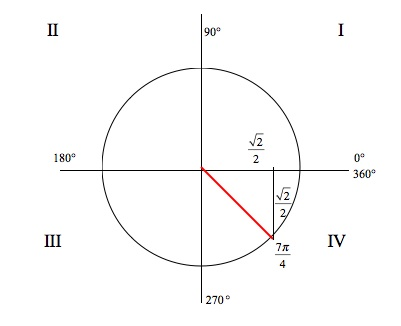• Looking at our picture, we see that [(7π)/4] is in quadrant IV. This will help us to solve for the sine and cosine values as well.
• Note that 315° is 45° from the x - axis so we can use the 45° − 45° − 90° triangle to find the sine and cosine.
• Recall that cosine is the x coordinate and sine is the y coordinate of [(7π)/4] on the unit circle
• cos [(7π)/4] = [(√2 )/2] and sin [(7π)/4] = − [(√2 )/2]
• Using the Mnemonic ASTC (All Students Take Calculus) helps to determine which values are positive in which quadrant
•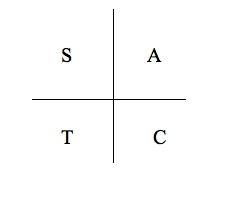• Since [(7π)/4] is in quadrant IV we know that cosine will be positive and sine will be negative
[(7π)/4] is in quadrant IV and cos [(7π)/4] = [(√2 )/2] and sin [(7π)/4] = − [(√2 )/2]
Convert [(4π)/3] to degrees, identify its quadrant, and find its cosine and sine.
• First convert [(4π)/3] to degrees by using the following formula: radian measure ×[(180°)/(π)] = degree measure
• [(4π)/3] ×[(180°)/(π)] = 4 ×60° = 240°
• Locate 240° on a unit circle (draw a picture). This will help you to determine the quadrant it is in and it will also help you to find the sine and cosine values.
•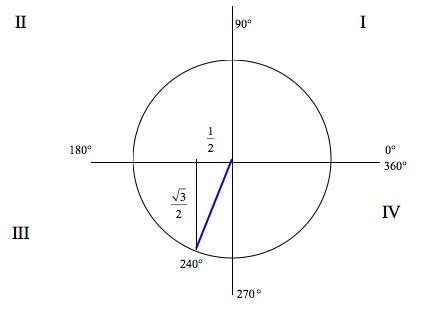• Looking at our picture, we see that 240° is in quadrant III. This will help us to solve for the sine and cosine values as well.
• Note that [(4π)/3] is [(π)/3] past the x - axis so we can use the 30° − 60° − 90° triangle to find the sine and cosine.
• Recall that cosine is the x coordinate and sine is the y coordinate of 240° on the unit circle
• cos 240° = − [1/2] and sin 240° = − [(√3 )/2]
• Using the Mnemonic ASTC (All Students Take Calculus) helps to determine which values are positive in which quadrant
•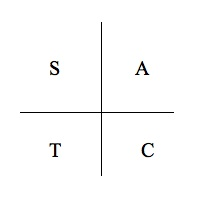• Since 240° is in quadrant III we know that tangent will be positive so sine and cosine will be negative
240° is in quadrant III and cos 240° = − [1/2] and sin 240° = − [(√3 )/2]
Convert 210° to radians, identify its quadrant, and find its cosine and sine.
• First convert 210° to radians by using the following formula: degree measure ×[(π)/(180°)] = radian measure
• 210° ×[(π)/(180°)] = [(210°π)/(180°)] = [(7π)/6]
• Locate [(7π)/6] on a unit circle (draw a picture). This will help you to determine the quadrant it is in and it will also help you to find the sine and cosine values.
•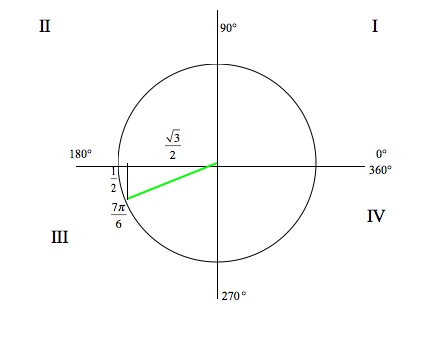• Looking at our picture, we see that [(7π)/6] is in quadrant III. This will help us to solve for the sine and cosine values as well.
• Note that 210° is 30° past the x - axis so we can use the 30° − 60° − 90° triangle to find the sine and cosine.
• Recall that cosine is the x coordinate and sine is the y coordinate of [(7π)/6] on the unit circle
• cos [(7π)/6] = − [(√3 )/2] and sin [(7π)/6] = − [1/2]
• Using the Mnemonic ASTC (All Students Take Calculus) helps to determine which values are positive in which quadrant
•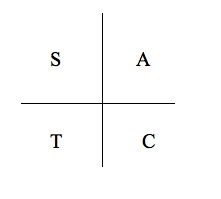• Since [(7π)/6] is in quadrant III we know that tangent will be positive so cosine and sine will be negative
[(7π)/6] is in quadrant III and cos [(7π)/6] = − [(√3 )/2] and sin [(7π)/6] = − [1/2]
Convert [(3π)/4] to degrees, identify its quadrant, and find its cosine and sine.
• First convert [(3π)/4] to degrees by using the following formula: radian measure ×[(180°)/(π)] = degree measure
• [(3π)/4] ×[(180°)/(π)] = 3 ×45° = 135°
• Locate 135° on a unit circle (draw a picture). This will help you to determine the quadrant it is in and it will also help you to find the sine and cosine values.
•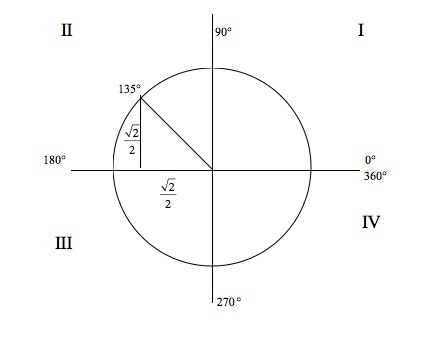• Looking at our picture, we see that 135° is in quadrant II. This will help us to solve for the sine and cosine values as well.
• Note that [(3π)/4] is [(π)/4] from the x - axis so we can use the 45° − 45° − 90° triangle to find the sine and cosine.
• Recall that cosine is the x coordinate and sine is the y coordinate of 135° on the unit circle
• cos 135° = − [(√2 )/2] and sin 135° = [(√2 )/2]
• Using the Mnemonic ASTC (All Students Take Calculus) helps to determine which values are positive in which quadrant
•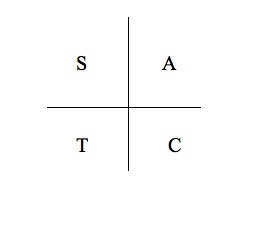• Since 135° is in quadrant II we know that sine will be positive and cosine will be negative
135° is in quadrant II and cos 135° = − [(√2 )/2] and sin 135° = [(√2 )/2]
Convert 150° to radians, identify its quadrant, and find its cosine and sine.
• First convert 150° to radians by using the following formula: degree measure ×[(π)/(180°)] = radian measure
• 150° ×[(π)/(180°)] = [(150°π)/(180°)] = [(5π)/6]
• Locate [(5π)/6] on a unit circle (draw a picture). This will help you to determine the quadrant it is in and it will also help you to find the sine and cosine values.
•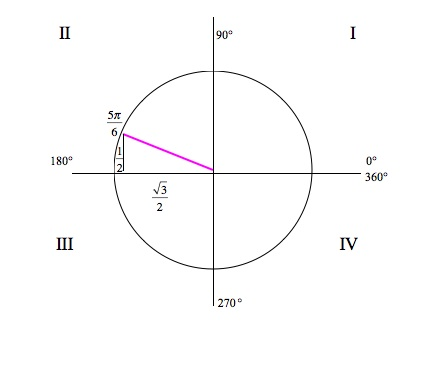• Looking at our picture, we see that [(5π)/6] is in quadrant II. This will help us to solve for the sine and cosine values as well.
• Note that 150° is 30° from the x - axis so we can use the 30° - 60° - 90° triangle to find the sine and cosine.
• Recall that cosine is the x coordinate and sine is the y coordinate of [(5π)/6] on the unit circle
• cos [(5π)/6] = − [(√3 )/2] and sin [(5π)/6] = [1/2]
• Using the Mnemonic ASTC (All Students Take Calculus) helps to determine which values are positive in which quadrant
•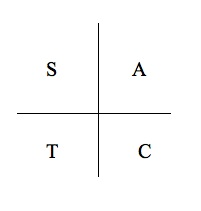• Since [(5π)/6] is in quadrant II we know that sine will be positive and cosine will be negative
[(5π)/6] is in quadrant II and cos [(5π)/6] = − [(√3 )/2] and sin [(5π)/6] = [1/2]
Evaluate sin([(3π)/4])
• Locate [(3π)/4] on a unit circle (draw a picture). This will help you to determine the quadrant it is in and it will also help you to find the sine value.
•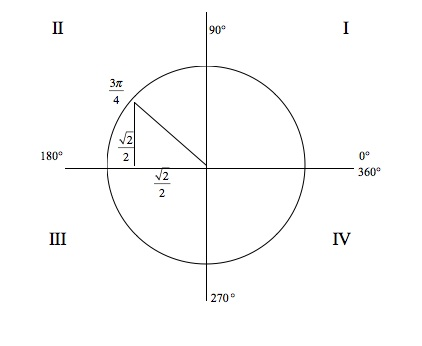• Looking at our picture, we see that [(3π)/4] is in quadrant II. This will help us to solve for the sine value.
• Note that [(3π)/4] is [(π)/4] from the x - axis so we can use the 45° − 45° − 90° triangle to find the sine value.
• Recall that sine is the y coordinate of [(3π)/4] on the unit circle
• sin [(3π)/4] = [(√2 )/2]
• Using the Mnemonic ASTC (All Students Take Calculus) helps to determine which values are positive in which quadrant
•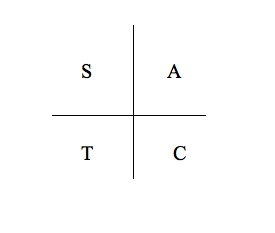• Since [(3π)/4] is in quadrant II we know that sine will be positive.
sin ([(3π)/4]) = [(√2 )/2]
Evaluate cos 330°
• Locate 330° on a unit circle (draw a picture). This will help you to determine the quadrant it is in and it will also help you to find the cosine value.
•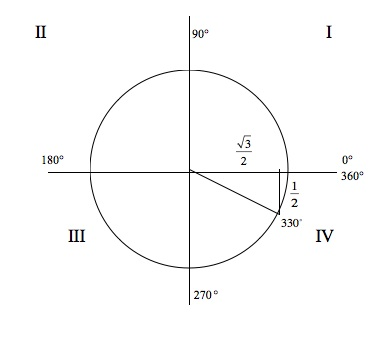• Looking at our picture, we see that 330° is in quadrant IV. This will help us to solve for the cosine value.
• Note that 330° is 30° from the x - axis so we can use the 30° - 60° - 90° triangle to find the cosine value.
• Recall that cosine is the x coordinate of 330° on the unit circle
• cos 330° = [(√3 )/2]
• Using the Mnemonic ASTC (All Students Take Calculus) helps to determine which values are positive in which quadrant
•• Since 330° is in quadrant IV we know that cosine will be positive.
cos 330° = [(√3 )/2]
Evaluate sin([(5π)/4])
• Locate [(5π)/4] on a unit circle (draw a picture). This will help you to determine the quadrant it is in and it will also help you to find the sine value.
•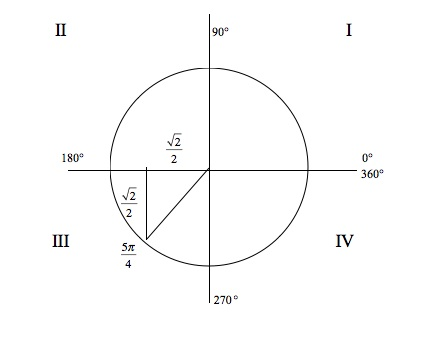• Looking at our picture, we see that [(5π)/4] is in quadrant III. This will help us to solve for the sine value.
• Note that [(5π)/4] is [(π)/4] past the x - axis so we can use the 45° − 45° − 90° triangle to find the sine value.
• Recall that sine is the y coordinate of [(5π)/4] on the unit circle
• sin [(5π)/4] = − [(√2 )/2]
• Using the Mnemonic ASTC (All Students Take Calculus) helps to determine which values are positive in which quadrant
•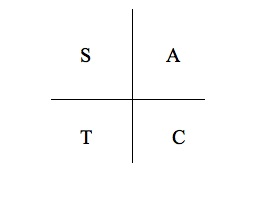• Since [(5π)/4] is in quadrant III we know that sine will be negative.
sin ([(5π)/4]) = − [(√2 )/2]
Identify all angles between 0 and 2π whose cosine is [1/2] in both degrees and radians. Identify which quadrant each is in.
• Recall that cosine is the x coordinate.
• Draw a unit circle and locate positive [1/2] along the x - axis. Draw a vertical line through that point. This will help you to identify the two points on the circle.
•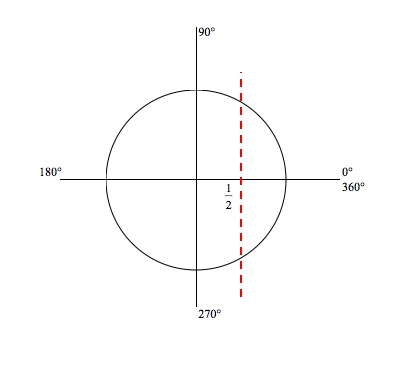• Connect the points on the circle to the origin. Notice that a 30° - 60° - 90° triangle was formed.
•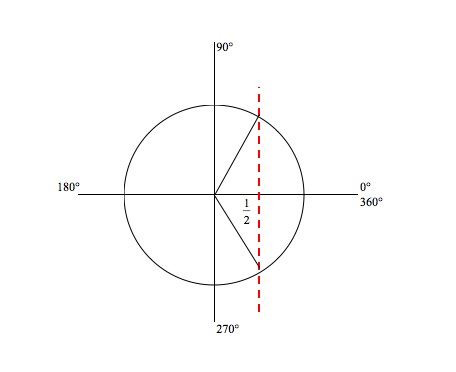• The first angle is 60° past 0° ⇒ 0° + 60° = 60°
• The second angle is 30° past of 270°⇒ 270° + 30° = 300°
 Angle 1 Angle 2 Degree 60° 300° Radian [(π)/3] [(5π)/3] Quadrant I IV
Identify all angles between 0 and 2π whose sine is − [(√3 )/2] in both degrees and radians. Identify which quadrant each is in.
• Recall that sine is the y coordinate.
• Draw a unit circle and locate − [(√3 )/2] along the y - axis. Draw a horizontal line through that point. This will help you to identify the two points on the circle.
•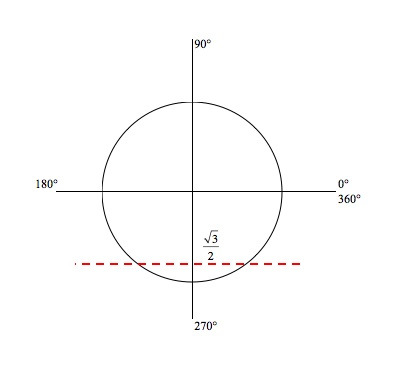• Connect the points on the circle to the origin. Notice that a 30° - 60° - 90° triangle was formed.
•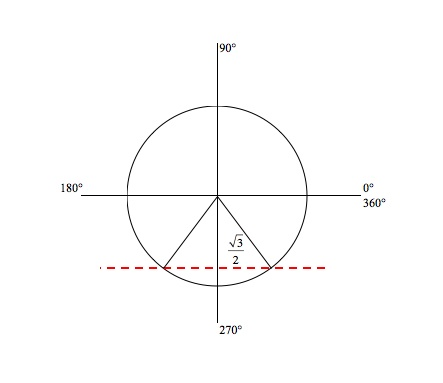• The first angle is 60° past 180° ⇒ 180° + 60° = 240°
• The second angle is 60° short of 360° ⇒ 360° − 60° = 300°
 Angle 1 Angle 2 Degree 240° 300° Radian [(4π)/3] [(5π)/3] Quadrant I IV

*These practice questions are only helpful when you work on them offline on a piece of paper and then use the solution steps function to check your answer.

Answer

### Sine and Cosine Values of Special Angles

Lecture Slides are screen-captured images of important points in the lecture. Students can download and print out these lecture slide images to do practice problems as well as take notes while watching the lecture.

• Intro 0:00
• 45-45-90 Triangle and 30-60-90 Triangle 0:08
• 45-45-90 Triangle
• 30-60-90 Triangle
• Mnemonic: All Students Take Calculus (ASTC) 5:21
• Using the Unit Circle
• New Angles
• Other Quadrants
• Mnemonic: All Students Take Calculus
• Example 1: Convert, Quadrant, Sine/Cosine 13:11
• Example 2: Convert, Quadrant, Sine/Cosine 16:48
• Example 3: All Angles and Quadrants 20:21
• Extra Example 1: Convert, Quadrant, Sine/Cosine
• Extra Example 2: All Angles and Quadrants

### Transcription: Sine and Cosine Values of Special Angles

Hi we are working out some examples of common values of sin and cos of common angles.0000

Remember what we learned, everything comes back to knowing those two key triangles.0007

There is the 45, 45, 90 triangle whose values are (root2)/2, (root 2)/2 and 1.0014

And then there is the 30, 60, 90 triangle whose values are 1/2, (root 3)/2 and 1.0025

If you remember those set of numbers, you can work out sin and cos of any common value anywhere on the unit circle.0038

That is what we are doing here, we have given the values 225 degrees, convert it to radians, let us start with that.0046

225 x pi/180 is equal to, well 225/180 simplifies down to 5/4, so that is 5pi/4.0053

Let us draw that on the unit circle and see where it lands.0069

My unit circle is 0, pi/2, which is the same as 90 degrees, pi radians is equal to 180 degrees, and 3pi/2 radians is 270 degrees, 2pi radians is equal to 360 degrees.0085

We have got 225 degrees or 5pi/4, 225 is between 180 and 270, in fact it is exactly half way between there because it is 45 degrees from either side.0105

It is right there, if you like that in terms of radians, 5pi/4 is just pi + pi/4, that is the angle we are looking at.0118

That is in the third quadrant, we found its quadrant, we converted it to radians, we want to find its cos and sin, that is the x and y coordinates.0133

Let me draw those in there, we want to figure out what those x and y coordinates are.0146

Look that is a common triangle and I remember what the values of those common triangles are.0150

That distance is (root 2 )/2, that distance is (root 2)/2.0157

I’m getting that because I remember this common 45, 45, 90 right triangle.0162

That means the sin and cos of both (root 2)/2 and we just need to figure out whether they are positive or negative.0171

All students take calculus, down there on the third quadrant only the tan is positive, both the sin and cos are negative.0188

Another way to remember that is just to remember that in the third quadrant both x and y values are negative.0201

Sin and cos of this angle are both negative (root 2)/2.0207

Again, what we are using over and over in these examples is these two key triangles.0220

The 45, 45, 90 triangle and the 30, 60, 90 triangle, you want to remember the values for those two triangles, (root 2)/2, (root 3)/2, and ½.0228

Remember those values and then when you have an angle that is in the other quadrants, it is just a matter of translating one of those triangles over there.0244

And then figuring out whether the sin and cos are positive and negative.0252

Let us try one more example here, we want to identify all the angles between 0 and 2 pi whose cos is –root 3/2.0000

I start by drawing my unit circle that is not quite straight, let me straighten that up a little bit.0010

Cos is – root 3/2, now the cos remember is the (x) value so I’m going to go on the (x) axis and I’m going to go to –root 3/2.0037

There it is.0053

That is –root 3/2 on the (x) axis and then I’m going to draw and see what angles I will get from that.0058

It looks like, remember that root 3/2 is one of my common values, that means that the y values are going to be ½.0073

I need to figure out which angles those are but that is one of my common values ½ root 3/2 that means that is a 30 degree angle, that is 60 and that is 30.0083

I just need to figure out what those angles are, if you remember we started 0, 90, 180, 270, and 360.0097

That first angle there is 30 degrees short of 180, the first angle is 150 degrees.0111

The second angle is 30 degrees past 180, so that is 210 degrees.0120

I have got my angles in degrees I will convert them into radians x pi/180 is equal to 5pi/6 to 10 x pi/180 is 7pi/6 radians.0127

I got those two angles in radians now, that is the first one 5pi/6, that is the second one 7pi/6.0151

And identify which quadrant each one is in, one of them is in the second quadrant, one of them is in the third quadrant, quadrant 2 and quadrant 3.0159

It all comes back to recognizing those common values, ½, square root of 3/2, square root of 2/2.0184

Once you recognize those common values, you can put these triangles in any position anywhere on the unit circle.0191

You just figure out where is your root 3/2, where is your ½, where is your root 2/2 and then you figure out which one is positive and which one is negative.0198

The whole point of this is you can figure out the sin and cos of any angle anywhere on the unit circle as long as it is a multiple of 30 or 45, or in terms of radians if it is a multiple of pi/6, pi/6, pi/4, pi/3.0209

You can figure out sin and cos of all these angles just by going back to those 3 common values and by figuring out whether their sin and cos are positive or negative.0226

Now you know how to find sin and cos of special angles, this is www.educator.com, thanks for watching.0238

Hi, these are the trigonometry lectures on educator.com.0000

Today we're going to learn about sine and cosine values of special angles.0003

When I say these special angles, there are certain angles that you really want to know by heart.0009

Those are the 45-45-90 triangle, and the 30-60-90 triangle.0014

Let me talk about the 45-45-90 triangle first.0022

I'll draw this in blue.0028

Here's a 45-45-90 triangle and I'm going to say that each side has length 1.0041

If each of the short sides has length 1, by the Pythagorean theorem, we can figure out that the long side, the hypotenuse, would have length square root of 2.0052

I'm going to scale this triangle down a little bit now.0066

I wanted to scale it down so the hypotenuse has length 1.0071

That means I have to divide all three sides by square root of 2.0075

If I scale this down, so the hypotenuse has length 1 that means the shorter sides has length 1 over the square root of 2, because I divided each side by square root of 2.0079

Then if you rationalize that, the way you learned in your algebra class, multiply top and bottom by square root of 2.0095

You get square root of 2 over 2, and square root of 2 over 2.0102

Those are very important values to remember because those are going to come up as sines and cosines of our 45-degree angles on the next slide.0113

First, I'd like to look also at the 30-60-90 triangle.0122

I have to do a little geometry to work this out for you.0129

I'm going to start with an equilateral triangle, a triangle where all three sides have 60 degrees.0133

I'm going to have assume that each side has length 2.0142

The reason I'm going to do that is because I'm going to divide that triangle in half.0145

If we divide that triangle in half, then we get a right angle here and each one of these pieces will have length 1.0150

Now, if I just look at the right hand triangle.0160

Remember that each one of the corners of the original triangle was 60 degrees.0169

That means that the small corner is 30 degrees, and I have a right angle here.0175

Now, the short side has length 1, the long side has length 2.0182

I'm going to figure out what the other side is using the Pythagorean theorem.0186

Let me call that x for now.0191

I know that x2 + 12 = 22, which is 4.0194

So, x2 = 4 - 1, which is 3.0202

So, x is the square root of 3.0209

That's where I got this relationship, 1, square root of 3, 2.0213

There's the 1, there's the square root of 3, and there's the 2.0219

Now, I'm going to turn this triangle on its side, and I want to scale it down.0223

Originally, it was 1, square root of 3, 2.0232

But again, I want to scale the triangle down so that the hypotenuse has length 1.0238

To do that, I have to divide everything by 2.0244

So the short side now has length 1/2.0247

The longer of the two short sides has length square root of 3 over 2.0251

Remember that's the side adjacent to the 30-degree angle.0258

That's the side adjacent to the 60-degree angle,0262

That's the right hand side.0264

These two triangles are very key to remember, in remembering all the sines and cosines.0267

In fact, if you can remember these lengths of these two triangles, you can work out everything else just from these two triangles.0273

Let me emphasize again, the 45-45-90 triangle, its sides have length 1, square root of 2 over 2, and square root of 2 over 2.0282

The 30-60-90 triangle, has sides of length 1, 1/2, and root 3 over 2.0297

Those are the values that you need to remember.0310

If you can remember those, you can work out all the sines and cosines you need to know for every trigonometry class ever.0312

Let's explore those a little bit.0321

We already figured out, let me draw a unit circle.0324

We know that sines and cosines occur as the x and y coordinates of different angles.0328

Well, if you're at 0...0338

Let me just draw in some key angles here, 0, here's 90, here's 45, here's 30, and here's 60.0341

If you're at 0 degrees, which is the same as 0 radians, then the cosine and sine, the x and y coordinates are just 1 and 0.0359

We already figured those out before.0368

The other easy one is the 90-degree angle up here.0370

We figured out that that's π/2 radians, and the cosine and sine are 0, and 1 there.0374

Now, the new ones, let me start with 45, because I think that one's a little bit easier.0380

The 45-degree angle, there it is right there.0386

We want to figure out what the x and y coordinates are because those give us the sine and cosine.0391

Well, we just figured out that a triangle that has 1 as its hypotenuse has square root of 2 over 2, as both its x and y sides.0397

That's where we get the square root of 2 over 2 as the cosine and sine of the 45-degree angle, also known as π/4 radians.0407

For the 30-degree angle, I'll do this one in blue.0418

The 30-degree angle, we have again, hypotenuse has length 1.0422

Remember, the length of the long side is root 3 over 2.0431

And the length of the short side is 1/2.0436

That's how you know the sine and cosine of the 30-degree angle or π/6.0439

The cosine, the x-coordinate, root 3 over 2, sine is 1/2.0447

The 60-degree angle, that's just the same triangle but it's flipped the other way so that the long side is on the vertical part and the short side is on the horizontal axis.0452

The short side is 1/2.0468

The long side is now the y-axis, that's root 3 over 2.0473

That's how we get 1/2 being the cosine of 60 degrees, root 3 over 2 being the sine of 60 degrees.0478

These values are really worth memorizing but you remember that you figure all out from those two triangles.0488

All you need to know is that one triangle has length 1, has hypotenuse 1, and sides root 2 over 2, that's the 45-45-90 triangle.0494

The other triangle has hypotenuse 1, and then the long side is root 3 over 2, short side is 1/2, that's the 30-60-90 triangle.0509

Just take that triangle and you flip it whichever way you need to, to get the angle that you're looking for.0526

These angles, these sines and cosines are the key ones to remember, root 2 over 2, root 3 over 2, and 1/2.0534

From that, what you're going to do is figure out the sines and cosines of all the other angles all over the unit circle.0546

Here's my unit circle.0573

We figured out all the sines and cosines of all the angles in the first quadrant.0575

All we have to do now is figure out all the sines and cosines of the angles in the other quadrants.0583

Let me draw them out.0589

But it's the same numbers every time.0590

All you have to do is figure out whether those numbers are positive or negative, and that just depends on which quadrant you're in.0593

All you have to do is remember those key numbers, root 2 over 2, root 3 over 2 and 1/2.0601

Then you're going to figure out which ones are positive in which quadrants.0607

Let me show you how you'll remember that, 1, 2, 3, 4.0613

Remember that the sine is the y value, and the cosine is the x value.0622

In the first quadrant, both the x's and y's are positive, and so sines and cosines are going to positive.0630

I'm going to write that as an a, which stands for all the values of everything is positive.0636

In the second quadrant, over here, the x values are negative, the y values are positive.0643

Now, the x values correspond to the cosines, the cosines are negative and the sines are positive.0653

I'm going to write as s here, for the sines being positive.0658

In the third quadrant, both x's and y's are negative.0667

X and y are both negative, that means cosines and sines are both negative.0669

Tangent, we haven't learned the details of tangent yet but we're going to learn later that tangent is sine over cosine.0675

Both sine and cosine are negative, that means sine over cosine is positive.0683

It turns out that tangent is positive down that third quadrant.0687

We'll learn the details of tangent later.0691

In the meantime, we'll just remember that tangent is positive in the third quadrant.0693

In the fourth quadrant here, that was the third quadrant that we just talked about, now we're moving on to the fourth quadrant.0698

The x's are positive now, the y's are negative.0705

That means the cosine is positive, but the sine is negative.0708

I'll list the cosine here because I'm listing the positive ones.0713

First quadrant, they're all positive.0718

Second quadrant, sines are positive.0720

Third quadrant, tangents are positive.0722

Fourth quadrant, cosines are positive.0724

The way you remember that is with this little acronym, All Students Take Calculus.0727

That shows you as you go around the four quadrants, All Students Take Calculus.0733

It shows you which ones are positive in each quadrant.0741

First quadrant are all positive.0744

Second quadrant sines are positive.0746

Third quadrant, tangents are positive.0748

Fourth quadrant, cosines are positive.0750

That's how you'll remember what the signs are in each quadrant.0753

That means the positive signs and the negative signs in each quadrant.0756

The numbers are just all these values that you've just memorized, root 3 over 2, root 2 over 2, and 1/2.0758

We'll do some practice finding sines and cosines of values in other quadrants, based on this table that we remember.0768

And these common values in common triangles that we remember.0775

Then we'll take those values, introduce some positive and negative signs, and we'll come up with the sines and cosines of angles in other quadrants.0780

Let's try some examples.0787

First example here is a 120 degrees, you want to convert that to radians, identify it's quadrant, and find it's cosine and sine.0791

First things first, let's convert it to radians, 120 times π/180 is 2π/3, because 120/180 is 2/3 so that's 2π/3 radians.0800

Identify it's quadrant.0806

Well, let me graph out my unit circle here.0814

There's 0, there's π/2, π, 3π/2, and then 2π.0820

Now, 2π/3 is between π/2 and π.0840

In fact, it's closer to π/2.0845

It's 2/3 of the way around from 0 to π.0848

If you like that in terms of degrees, π is 180 degrees, and so 2π/3 is 2/3 of the way over to 180.0852

Now, we're going to find cosine and sine.0862

Let me show you how to do this.0867

You draw a triangle here.0868

Remember, we're looking for the x and y coordinates.0870

Draw a triangle here.0873

That's a 30-60 triangle.0875

This is 60, that's a 60-degree angle.0877

That's a 30-degree angle.0887

We know what the lengths of these different sides are.0891

We know that the long side there is root 3 over 2 and the short side there is 1/2.0897

We know, remember, the cosines and sine are the x and y coordinates.0907

The cosine of 120 or 2π/3 is 1/2, except that we're going to have to check whether that's positive or negative.0910

Remember All Students Take Calculus.0930

In the second quadrant, only the sine is positive, so the cosine must be negative.0935

The sine of 2π/3, the y value is root 3 over 2.0942

In the second quadrant, sines are positive, so that's positive.0950

Our cosine and sine are -1/2 and root 3 over 2.0954

If you didn't remember the All Students Take Calculus thing, you can also just work it out once you know what quadrant it's in.0958

It's in quadrant 2 and we know there that the x coordinates are negative, and the y coordinates are positive.0964

The cosine must be negative and the sine must be positive.0974

The whole point of this is that you only really need to memorize the values of the triangles, root 2 over 2, root 3 over 2 and 1/2.0981

Once you know those basic triangles, you can work out what the sines and cosines are in any different quadrant just by drawing in those triangles and then figuring out which ones have to be positive, and which ones are to be negative.0991

Let's try another one.1005

This one is converting 5π/3 radians to degrees, identifying it's quadrant, and finding its cosine and sine.1009

5π/3 times 180/π, the π's cancel, and the we have 5/3 of 180, 180 over 3 is 80, so this is 5 times 60 is 300 degrees.1018

Let's try and find that in the unit circle.1040

We have 0, π/2 which is 90, π which is 180, 3π/2 which is 270, and 2π which is the same as 360 degrees.1053

Now, 5π/3, that's bigger than π and that's smaller than 2π.1074

In fact, that's π + 2π/3.1080

That's π, which is right here, plus 2π/3.1090

So, 2/3 the way around from π to 2π.1099

There it is right there.1103

That's in the fourth quadrant.1104

So, we figured out what quadrant it's in.1108

If you like degrees better, 300 degrees is a little bigger than 270, in fact, it's 30 degrees past 270, and 60 degrees short of 360.1110

That's how you know that that angle is in that quadrant.1121

Now we have to find its cosine and sine.1126

That's the x and y coordinates.1128

We set up our triangle there.1134

We already know that that's a 60-degree angle, because it's 60 degrees short of 360.1137

That's a 30-degree angle and we just remember our common values.1142

The horizontal value, that's the short one, that's 1/2, that's the long one, root 3 over 2.1147

We know our values are going to be 1/2 and root 3 over 2, we'll just have to figure out which one's positive and which one's negative.1154

I know my cosine of 5π/3 is going to be either positive or negative 1/2.1162

The sine of 5π/3 is positive or negative root 3/2.1171

Remember All Students Take Calculus.1179

Down there in the fourth quadrant, the cosine is positive and the sine is negative.1184

If you don't remember All Students Take Calculus, you just look that you're in the fourth quadrant, x coordinates are positive, y coordinates are negative.1189

You know which one's positive or negative.1199

All you have to remember are those key values, 1/2, root 3 over 2, root 2 over 2.1202

Remember those key values for the key triangles.1209

Then it's just a matter of drawing the right triangle in the right place and figuring out which one is positive, and which one is negative.1212

Let's try another one.1219

This one is kind of tricky.1223

This one's going to be challenging us to go backwards from the sine.1224

We have to find all angles between 0 and 2π whose sine is -1/2.1229

This is kind of foreshadowing the arc sine function that we'll be studying later on and one of the later lectures.1242

In the meantime, the sine is -1/2.1249

Remember now, the sine is the y-coordinate.1252

We want things whose y coordinates are -1/2.1256

I'm going to draw -1/2 on the y-axis, -1/2.1259

I'm going to look for all angles whose y-coordinate is -1/2.1266

Look, there's one right there.1272

And there's one right there.1276

I'm going to draw those in.1277

I'm going to draw those triangles in.1280

I know now that if we have a vertical component of 1/2, the horizontal component has to be root 3 over 2.1289

That's because we remember those common triangles, 1/2, root 3 over 2, root 2 over 2.1298

We're going to figure out what those angles are.1307

I know that's a 30-degree angle.1312

I know that that is 180.1318

The whole thing is 210 degrees.1322

I know that that is 30.1330

I know that that must be 60.1334

Remember, this is 270 degrees down here.1337

We have 270 degrees plus 60 degrees.1342

This is getting a little messy, so I'm going to redraw it over here.1345

That's the angle we're trying to chase down here.1357

We know that's 60.1360

That much is 270.1362

So, 270 plus 60 is 330 degrees.1366

Those are the two angles that we're after, 210 degrees, 330 degrees.1372

Let me convert those into radians.1378

If you multiply that by π/180 then that's equal to...1382

Let's see, that's 7π/6 radians.1390

This one times π/180 is equal to 11π/6 radians.1397

We've got our two angles in degrees and radians.1409

The quadrants, the first one was in the third quadrant, quadrant 3.1413

The second one was in the fourth quadrant, quadrant 4.1420

Those are the two angles in both degrees and radians that had a sign of -1/2.1426

Their y value was -1/2.1436

What this comes down to is knowing those common values, 1/2 root 3 over 2, root 2 over 2.1442

Once you know those common values, it's a matter of looking at the different quadrants and figuring out whether the x and y values are positive or negative.1454

In this case, we had the sine, sine remember is the y-coordinate.1461

Since it was negative, we knew that we had to be at -1/2 on the y-axis.1468

We found -1/2 on the y-axis, drew in the triangles, recognized the 30-60 triangles that we've been practicing and then we were able to work out the angles.1473

We'll try some more examples of that later.1484

Please sign in to participate in this lecture discussion.

Resetting Your Password?
OR

### Start Learning Now

Our free lessons will get you started (Adobe Flash® required).
Get immediate access to our entire library.

### Membership Overview

• Available 24/7. Unlimited Access to Our Entire Library.
• Search and jump to exactly what you want to learn.
• *Ask questions and get answers from the community and our teachers!
• Practice questions with step-by-step solutions.
• Download lecture slides for taking notes.
• Track your course viewing progress.
• Accessible anytime, anywhere with our Android and iOS apps.